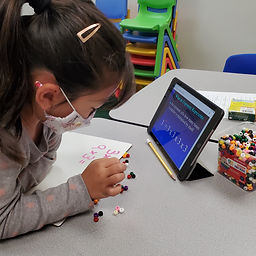## Ms. Raejean

### Target 1​

###### Lesson Type:

New

Number Operation

:

Computation

Evaluate arithmetic expressions that include an exponent.

###### 1:

Understand that an exponent indicates the number of times that a base is multiplied by itself.

###### 2:

Write a repeating multiplication equation that is equivalent to a given exponent.

3rd

###### Vocabulary:

Exponents, Base, Multiplication

Activities:

Students learned about exponents, which indicate how many times the base needs to be multiplied by itself. Students wrote the exponent as a repeated multiplication equation. Ex. 3⁴ = 3x3x3x3. Then students computed one of the exponents using beads to represent it. Ex. 3⁴ = 3x3x3x3.

• 27 groups of 3 beads### Home Exploration

###### Guiding Questions:## Absent Students:

### Target 2

:

###### 1:

Create the reflection of a given figure.

###### 2:

Create a reflection on a coordinate plane.

3rd

###### Vocabulary:

Symmetry, Reflection(al), Flip, Line of Symmetry

Activities:

Students shared what they know about symmetry. We discussed reflectional symmetry. The shape is flipped over the line of symmetry to complete the missing half or copy a mirror image of the shape. Students used a mirror to see the reflection of a given shape and drew the missing part.### Home Exploration

###### Guiding Questions:### Target 3

:

###### 1:

Identify the center point of a shape.

###### 2:

Rotate a given figure at 90-degree intervals around its center point.

3rd

###### Vocabulary:

Symmetry, Rotation(al), Turn, 90 Degrees, 360 Degrees, Line of Symmetry

Activities:

Students continued learning about symmetry. We discussed rotational symmetry. A shape is turned in intervals to complete a 360 degree turn. Students drew the shape in its “new” position after a 90 degree rotation. Then, students created images with rotational symmetry.### Home Exploration